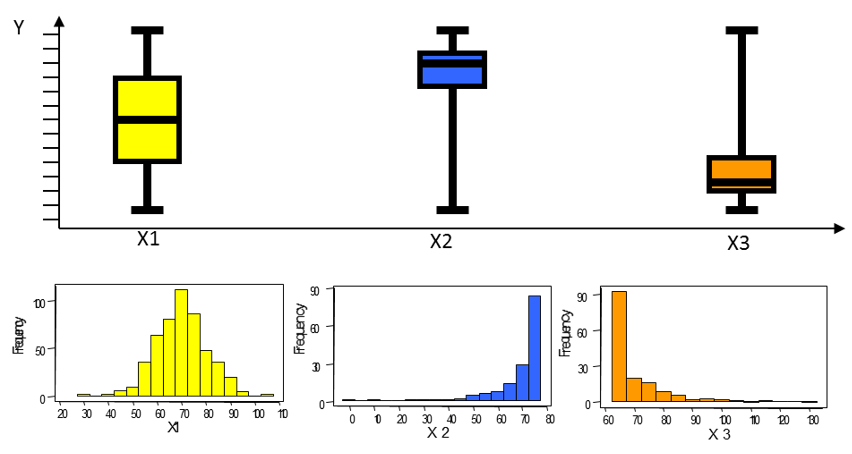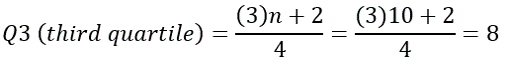top of page
Buscar
• edsonmontoro

# Box Plot Versatility [EN]

I am a fan of this tool, it is very simple, easy to create and to interpret, as it is very visual. It has many practical applications.  Enjoy!

PS: You can check the article here by the blog or if you prefer to download the article by clicking here.

## Box Plot Versatility

Author: Edson R. Montoro

The Box Plot, created by John Tukey, has numerous applications besides being a very useful tool and easy to construct and interpret.

There are many variations of Box Plot, because it is a simple and very visual tool sometimes there are certain exaggerations in putting a lot of information about it. It is recommended that it be as simple as possible, with the information needed to really show only what you want; without visual pollution, otherwise it loses all its strength.

In this article we will show the most basic types of Box Plot and some application examples.

### 1. Simple Box Plot

Basically, the Box Plot (Figure 1) shows the distribution of experimental results and is composed of the following values: “Lowest Value”, “1st Quartile”, “2nd Quartile” (or Median), “3rd Quartile” and “Highest Value”.Figure 1 - Interpretation of the simple Box Plot.

These statistics are easily calculated by Formulas 1, 2 and 3, which produce the position of the respective statistic, which has the corresponding experimental result.Normally when we have few measurements of a random variable, we cannot build a Histogram (at least 50 data are required for a good Histogram); to visualize the distribution of these results, we use the Box Plot.

A comparison between the Box Plot and the Histogram can be seen in Figure 2.Figure 2 - Comparison of Box Plot with Histogram.

There are applications that already build the Box Plot automatically, such as Action, Minitab, Statgraphics, JMP and others; but you can build an Excel spreadsheet for the necessary calculations.

To calculate the first, second and third quartiles (Q1, Q2 or median, Q3); you must first sort the data in ascending order and then apply the formulas (1), (2) and (3) seen above.

We can see an example of the calculations using the data of two random variables with 10 values each (n1 = n2 = 10) presented in Table 1.

Table 1 - Variable Response: processing time (min).To calculate the median (second quartile) of both X1 and X2, Formula 2 is used:Since the position is 5.5th, the value of the Median must be between the 5th and the 6th, which according to the example: for X1 are respectively 243 and 251; then the Median will be the average between these two values, 247. For X2, it will be the average between 188 and 192, which is 190.

The 1st Quartile calculated by Formula 1:is the value that occupies the 3rd position, which in the example shown, for X1 is 207 and for X2, 145. For the 3rd Quartile, calculated by Formula 3:is the value of the 8th position, which for X1 is 272 and for X2 is 228. These results can be seen in figure 3.Figure 3 - Comparison of variables with Box Plot.

### 2. Notched Box Plot

The notched Box Plot (Figure 4) includes the 95% Confidence Interval information for the Median; which means that it is an estimate of the median per range, that is, the actual median value with 95% certainty must be within this range.Figure 4 - Interpretation of the notched box plot.

The use of this type of Box Plot is in statistical comparisons as if it were a “visual” Hypothesis Test. If the chamfers of two or more Box Plots match, we can say that there is no significant difference between the medians at a significance level of 5%.

If the variables can be considered as a good approximation for the Normal distribution model, we can approach this conclusion also for the averages.

Here are three examples of notched Box Plot applications.

### 2.1. Example 1: Comparison of variability and central tendency of various equipment.

As shown in Figure 5, there is no significant difference between equipment B and C, as there is a coincidence between the respective confidence interval, whereas A is different from these two with respect to the median since the confidence interval does not match.Figure 5 – Comparison using Box Plot.

As for variability, it is not possible to say that there is a significant difference between the three pieces of equipment, because the Box Plots heights are very similar to each other.

## 2.2. Example 2: Comparison of the performance of a random variable over time.

As shown in Figure 6, the variability decreased significantly, it is visible that month after month the height of the Box Plot, showing the experimental values decreased. In January, the range varies from approximately 1 to 15, while in April it is from 7 to 9.Figure 6 – Performance comparison using Box Plot.

## 2.3. Example 3: process monitoring.

This example is very interesting, in a chemical plant, the operators performed various level measurements and whenever this was greater than a pre-defined threshold (in this case 17 cm), they had to perform a rather bulky manual task.

For lack of guidance on the importance of controlling this process variable, whenever the level was greater than 17 cm, some operators noted the measurement value as 17 cm, and left the task for the next shift. This led to uncontrolled process, generating waste and impacting the control of other process variables.

Data from one period were plotted using Box Plot, and it was noted that most measurements were 17 cm or smaller, with few values greater than 17 cm; This can be easily seen in the Box Plots in Figure 7.Figure 7 – Problems identification using the Box Plot Technique.

After this analysis a Kaizen was made, involving some operators to improve the task and making it simpler. After the change, all operators underwent training to clarify the importance of controlling this process variable. Obviously, the waste was eliminated, and the gains were computed.

# 3. Outliers Detection

Another important application of Box Plot is the detection of outliers, that is, a strange value that probably does not belong to the population.

The distance between the first and third quartile is called the Interquartile Range (IQR) and contains almost 50% of the observed data. If a value exceeds 1.5 times this value (IQR), up or down, it may be considered as an outlier; see the example in Figure 8.Figure 8 - Outlier Identification using Box Plot.

The rationale of this technique is that considering this range of ± 1.5xRIQ, that is (Q1 - 1.5xIQR) to (Q3 + 1.5xIQR) practically corresponds to the Control Limits of a SPC (Statistical Process Control) control chart, as we can see in Figure 9.Figure 9 - Rationale of Outliers identification using Box Plot.

It is worth remembering that one cannot simply eliminate an outlier, we must find out its cause before any decision. Much can be learned about your process by identifying and analyzing outliers.

## References

(1) McGill, Robert; Tukey, John W.; Larsen, Wayne A.; Variations of Box Plots. The American Statistician, vol. 32 (1): pp. 12–16, February, 1978.